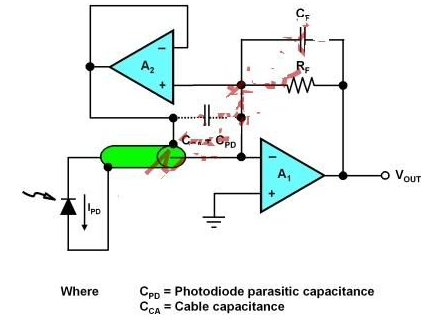# How to use photo sensing circuits to reduce photodiode bandwidth and noise effects

Photodiodes convert a fundamental physical phenomenon (light) into an electrical form (current). The design engineer systematically converts the photodetector current into a usable voltage, making the processing of the photodiode signal easy to control. There are many ways to deal with the problem of optical sensing circuits, but I have encountered a special problem. How to use a circuit that can reduce the photodiode bandwidth and noise effects remotely or through a large parasitic capacitance.

A typical optical sensing system circuit has a photodiode, operational amplifier, and feedback resistor/capacitor pair at the front end. This article begins with the circuit described in the previous article. In this circuit, photodiodes, amplifiers, and feedback capacitors limit the bandwidth of the circuit.

The amplifier input has a large input capacitance when using a large parasitic capacitance or at a long distance to perform light sensing through a photodiode. This increase in capacitance increases the noise gain of the circuit unless you increase the feedback capacitance of the amplifier. If the feedback capacitance (C F ) increases, the bandwidth of the circuit decreases.

To solve this problem, you can use a bootstrap circuit (see Figure 1). Photodiodes with lower diode capacitance do not benefit from this circuit. The unity gain buffer A 2 removes the cable capacitance and the photodiode parasitic capacitance generated by the transimpedance amplifier A1 input.Figure 1: Bootstrap diode capacitance and cable capacitance out of the transimpedance design problem.

When making this circuit design, the choice of A 2 amplifier type can be slightly relaxed. Only four performance specifications are more important. These design principles include choosing an amplifier with low input capacitance, low noise, and a higher bandwidth than A 1 and low output impedance.

In this design, the input capacitance of A 2 is the only capacitor that acts in the AC transfer function of the transimpedance system. The buffer input capacitor replaces the sum of the A 1 input capacitance, the cable capacitance, and the photodiode parasitic capacitance. A good rule of thumb is C A2 "(CA1 + CCA + CPD), where CA1 and CA2 are the sum of their input differential and common mode capacitance.

With this design, you can swap one noise problem (A 1) with another (A 2). The unity gain buffer eliminates the noise effects of A1. A good rule of thumb is to make A 2 noise "= A 1 .

The difference between the input signal and the output signal in the system is reduced after hitting the cable/diode capacitance. You can maintain this low signal difference by selecting A 2 with a larger bandwidth than A 1 and keeping the output impedance of A 2 low. The A 2 gain roll-off sets the upper limit for bandwidth improvement so that the bandwidth relationship between these amplifiers is equal to A 2-BW 》 A 1-BW . This circuit requires stable optimization when you balance the C F and A 2 input capacitors.